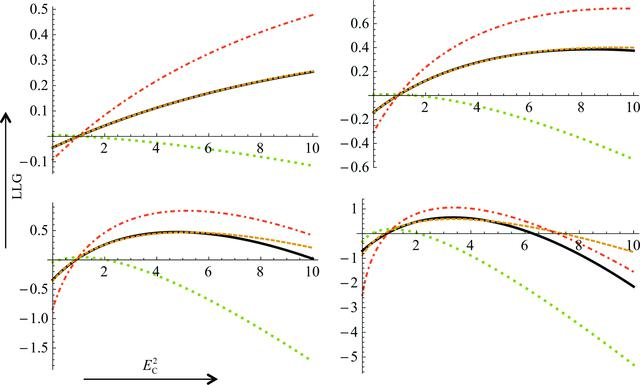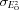disable zoom     view article Figure 4 Comparisons of different approximations to the exact LLG for the acentric case as the quality of the model is varied. All four plots represent the case shown in the upper left line plot of Fig. 3, in which EO2 = 3.0 and= 1.6. The exact probability distribution as a function of EC2 is shown as a black line, the LLGI approximation as a dashed orange line, the inflated-variance Rice-function approximation with French and Wilson estimates of the amplitude and its standard deviation as a dotted green line and the inflated-variance Rice-function approximation with estimates by simple variable transformation of the amplitude and its standard deviation as a dashed–dotted red line. The values of σA across the plots are 0.3 on the upper left, 0.5 on the upper right, 0.7 on the lower left and 0.9 on the lower right.STRUCTURALBIOLOGY
ISSN: 2059-7983
Volume 72| Part 3| March 2016| Pages 375-387